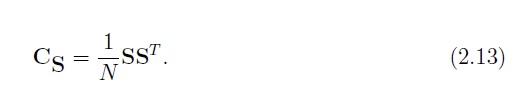## Principal Component Analysis

Principal component analysis (PCA) is a dimensionality reduction technique that attempts to recast a dataset in a manner that nds correlations in data that may not be evident in their native basis and creates a set of basis vectors in which the data has a low dimensional representation. PCA works by producing a set of vectors that point along the direction of maximum variance while being simultaneously orthogonal to each other. These are called the principal components. The first principal component vector is parallel to the direction of maximum variance. The second principal component points in the direction of maximum variance that is not explained by the first principal component. And so on. Imagine one has a dataset S which consists of N spectra with N spectral channels. Instead of looking at the data as just intensity versus spectral channel, PCA attempts to construct a set of new vectors (also called features) that show as much variation in the spectra as possible. In other words, first direction (principal component) is used to recast the data to look as different (uncorrelated) as possible. This allows us to discriminate the data, as best we can with just one direction. The second principal component is the direction that provides the second most ability to discriminate the data, and so on. Now I will discuss some of the math behind PCA. The covariance matrix is dened asEach element in the covariance matrixis the covariance of thespectrumand the nth spectrum.A large covariance means they look alike and therefore are dicult to disambiguate. Geometrically, it means thatandtend to point in the same direction (assuming their length is approximately the same). If the entire collection of spectra S were mutually orthogonal, being able to tell one spectrum apart from another would be easy. You would just have a collection of spikes at different spectral channels. The covariance matrix in this case would be a diagonal matrix. Therefore, it is desirable to have covariance matrices that are diagonal matrices since they indicate low correlation between the datasets and improves one’s ability to distinguish between the data.Since there is typically some redundancy between spectra, the off-diagonal elements of the covariance matrix will be non-zero. PCA nds a basis in which the covariance matrix is diagonalized. In this basis, the data is uncorrelated.where Y is the data projected onto the principal component basis. The rows of matrixare the principal components. The covariance of the projected datais a now diagonal matrix. Indeed, the principal components are the optimal way to discriminate the spectra in the dataset . It turns out the principal components are the eigenvectors of the covariance matrixare the principal components . Note that each eigenvector has an associated eigenvalue. The eigenvalues are also informative because the relative magnitude of the eigenvalue can tell us how many principal components are really needed to discriminate all of the spectra . The magnitude of the eigenvalue tells us how well useful the associated eigen vector is at discriminating the data. Since the full set of principal components forms a basis, each spectra s in S can be written as a superposition of principal components without any errorWhereis a scalar coeffcient value associated with eigenvector. In many cases, only a few of the First principal components are needed in the summation to approximate the original data well,.

This is another reason why PCA is so useful. It can be used as a way to perform dimensionality reduction. In other words, seemingly complicated data can be summarized by only a few principal components by exploiting the correlations between the data. This concept is analogous to lossy compression in signal processing. Simply project the data onto the First several principal components associated with the largest M eigenvalues. The data now has an approximately sparse representation.

https://search.proquest.com/openview/4c390c5e298854ed9383815aca5431c0/1?pq-origsite=gscholar&cbl=18750&diss=y